### Find Schools

Study Area & Zipcode

Mathematician

# What Do Mathematician Do?

Job Description: Conduct research in fundamental mathematics or in application of mathematical techniques to science, management, and other fields. Solve problems in various fields using mathematical methods.

## Mathematician Responsibilities

• Apply mathematical theories and techniques to the solution of practical problems in business, engineering, the sciences, or other fields.
• Design, analyze, and decipher encryption systems designed to transmit military, political, financial, or law-enforcement-related information in code.
• Perform computations and apply methods of numerical analysis to data.
• Conduct research to extend mathematical knowledge in traditional areas, such as algebra, geometry, probability, and logic.
• Address the relationships of quantities, magnitudes, and forms through the use of numbers and symbols.
• Develop mathematical or statistical models of phenomena to be used for analysis or for computational simulation.

## Mathematician Skills

These are the skills Mathematicians say are the most useful in their careers:

Mathematics: Using mathematics to solve problems.

Reading Comprehension: Understanding written sentences and paragraphs in work related documents.

Complex Problem Solving: Identifying complex problems and reviewing related information to develop and evaluate options and implement solutions.

Critical Thinking: Using logic and reasoning to identify the strengths and weaknesses of alternative solutions, conclusions or approaches to problems.

Active Learning: Understanding the implications of new information for both current and future problem-solving and decision-making.

Active Listening: Giving full attention to what other people are saying, taking time to understand the points being made, asking questions as appropriate, and not interrupting at inappropriate times.

• Research Scientist
• Computational Physicist
• Emerging Solutions Executive
• Computational Mathematician
• Cryptographic Vulnerability Analyst

## Are There Job Opportunities for Mathematicians?

In the United States, there were 3,100 jobs for Mathematician in 2016. New jobs are being produced at a rate of 29% which is above the national average. The Bureau of Labor Statistics predicts 900 new jobs for Mathematician by 2026. Due to new job openings and attrition, there will be an average of 300 job openings in this field each year.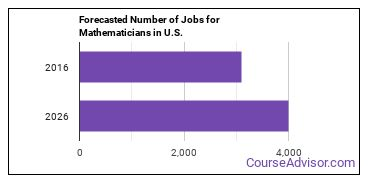The states with the most job growth for Mathematician are Idaho, New Jersey, and Virginia. Watch out if you plan on working in Maryland, Washington, or Pennsylvania. These states have the worst job growth for this type of profession.

## What is the Average Salary of a Mathematician

Mathematicians make between \$57,150 and \$160,550 a year.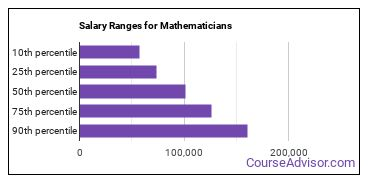Mathematicians who work in District of Columbia, Virginia, or Washington, make the highest salaries.

### Below is a list of the median annual salaries for Mathematicians in different U.S. states.

State Annual Mean Salary
California \$100,000
District of Columbia \$133,870
Florida \$87,820
Illinois \$103,220
Maryland \$115,940
New Jersey \$117,060
New Mexico \$106,790
New York \$84,670
Ohio \$86,280
Pennsylvania \$76,250
Rhode Island \$90,640
Virginia \$121,240
Washington \$123,380

## Tools & Technologies Used by Mathematicians

Below is a list of the types of tools and technologies that Mathematicians may use on a daily basis:

• Microsoft Excel
• Microsoft Word
• Microsoft Office
• JavaScript
• Microsoft PowerPoint
• Microsoft Outlook
• Web browser software
• Python
• Microsoft Access
• MySQL
• Structured query language SQL
• The MathWorks MATLAB
• SAS
• Linux
• Microsoft Visual Basic
• Extensible markup language XML
• IBM SPSS Statistics
• UNIX
• PHP: Hypertext Preprocessor

## Becoming a Mathematician

Individuals working as a Mathematician have obtained the following education levels: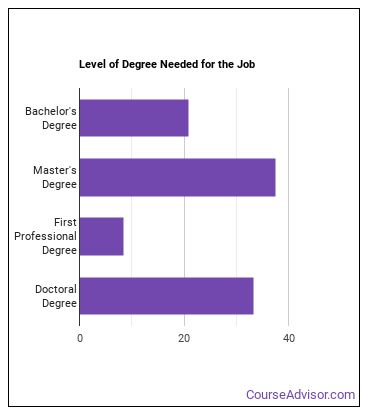## How many years of work experience do I need?## Where Mathematicians Are Employed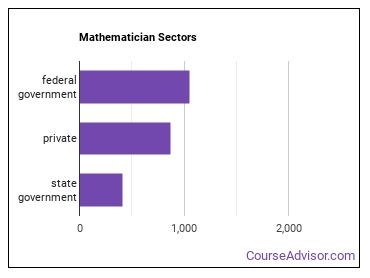Mathematicians work in the following industries: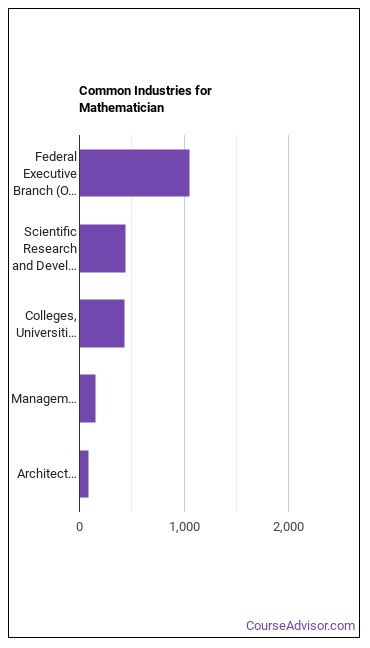Image Credit: Wallpoper via Public domain

More about our data sources and methodologies.

## Find Schools Near You

Our free school finder matches students with accredited colleges across the U.S.